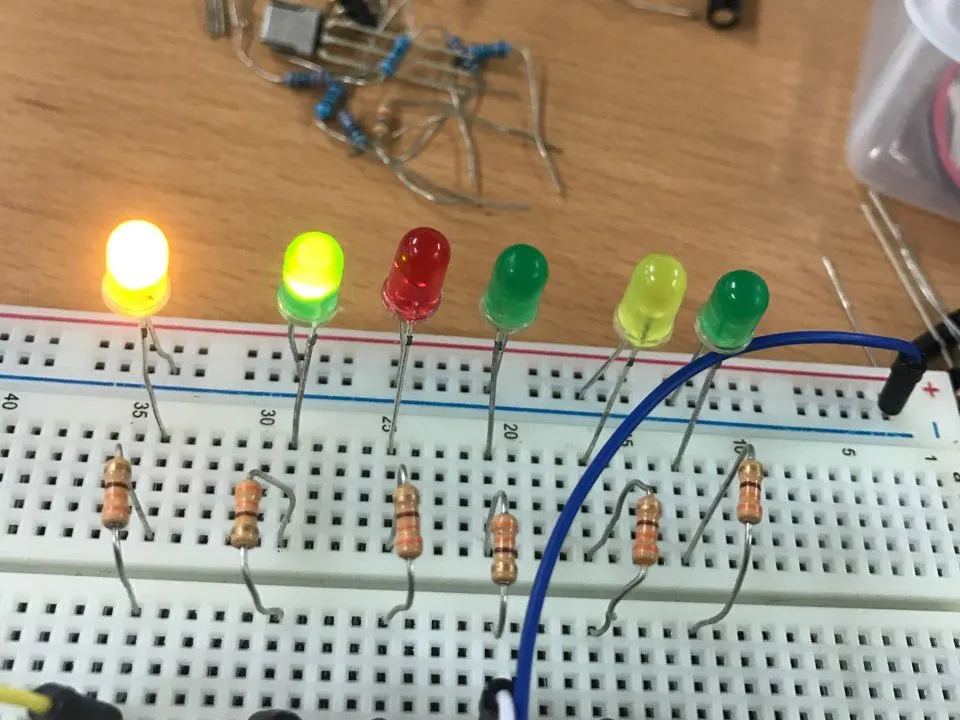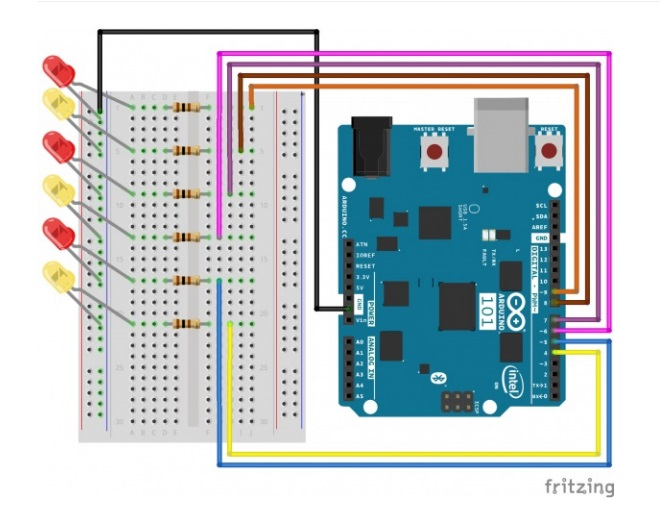Project in progress# Driving Multiple LEDs © GPL3+

I used coding to make several leds glow.

• 472 views
• 1 comment
• 3 respects

## Components and supplies

I tried coding many LEDs blinking

As shown picture,

Using wires, I connected leds with resistors at breadboard.

Leds began to glow as a result of connection.

## Code

```/*
SparkFun Inventor's Kit
Example sketch 04

MULTIPLE LEDs

Make six LEDs dance. Dance LEDs, dance!

This sketch was written by SparkFun Electronics,
with lots of help from the Arduino community.
This code is completely free for any use.
Visit http://learn.sparkfun.com/products/2 for SIK information.
Visit http://www.arduino.cc to learn about the Arduino.
*/

// To keep track of all the LED pins, we'll use an "array".
// An array lets you store a group of variables, and refer to them
// by their position, or "index". Here we're creating an array of
// six integers, and initializing them to a set of values:
int ledPins[] = {4, 5, 6, 7, 8, 9};

void setup()
{
//create a local variable to store the index of whcih pin we want to control
int index;

// For the for() loop below, these are the three statements:

//   1. index = 0;    Before starting, make index = 0.
//   2. index <= 5;   If index is less or equal to 5, run the following code
//   3. index++   Putting "++" after a variable means "add one to it".

// When the test in statement 2 is finally false, the sketch
// will continue.

// This for() loop will make index = 0, then run the pinMode()
// statement within the brackets. It will then do the same thing
// for index = 2, index = 3, etc. all the way to index = 5.

for (index = 0; index <= 5; index++)
{
pinMode(ledPins[index], OUTPUT);
}
}

void loop()
{
// This loop() calls functions that we've written further below.
// We've disabled some of these by commenting them out (putting
// "//" in front of them). To try different LED displays, remove
// the "//" in front of the ones you'd like to run, and add "//"
// in front of those you don't to comment out (and disable) those
// lines.

// Light up all the LEDs in turn
oneAfterAnotherNoLoop();

// Same as oneAfterAnotherNoLoop, but less typing
//oneAfterAnotherLoop();

// Turn on one LED at a time, scrolling down the line
//oneOnAtATime();

// Light the LEDs middle to the edges
//pingPong();

// Chase lights like you see on signs
//marquee();

//randomLED();
}

/*
oneAfterAnotherNoLoop()
This function will light one LED, delay for delayTime, then light
the next LED, and repeat until all the LEDs are on. It will then
turn them off in the reverse order.
*/

void oneAfterAnotherNoLoop()
{
// time (milliseconds) to pause between LEDs
int delayTime = 100;

// turn all the LEDs on:

digitalWrite(ledPins, HIGH);  //Turns on LED #0 (pin 4)
delay(delayTime);                //wait delayTime milliseconds
digitalWrite(ledPins, HIGH);  //Turns on LED #1 (pin 5)
delay(delayTime);                //wait delayTime milliseconds
digitalWrite(ledPins, HIGH);  //Turns on LED #2 (pin 6)
delay(delayTime);                //wait delayTime milliseconds
digitalWrite(ledPins, HIGH);  //Turns on LED #3 (pin 7)
delay(delayTime);                //wait delayTime milliseconds
digitalWrite(ledPins, HIGH);  //Turns on LED #4 (pin 8)
delay(delayTime);                //wait delayTime milliseconds
digitalWrite(ledPins, HIGH);  //Turns on LED #5 (pin 9)
delay(delayTime);                //wait delayTime milliseconds

// turn all the LEDs off:

digitalWrite(ledPins, LOW);   //Turn off LED #5 (pin 9)
delay(delayTime);                //wait delayTime milliseconds
digitalWrite(ledPins, LOW);   //Turn off LED #4 (pin 8)
delay(delayTime);                //wait delayTime milliseconds
digitalWrite(ledPins, LOW);   //Turn off LED #3 (pin 7)
delay(delayTime);                //wait delayTime milliseconds
digitalWrite(ledPins, LOW);   //Turn off LED #2 (pin 6)
delay(delayTime);                //wait delayTime milliseconds
digitalWrite(ledPins, LOW);   //Turn off LED #1 (pin 5)
delay(delayTime);                //wait delayTime milliseconds
digitalWrite(ledPins, LOW);   //Turn off LED #0 (pin 4)
delay(delayTime);                //wait delayTime milliseconds
}

/*
oneAfterAnotherLoop()

This function does exactly the same thing as oneAfterAnotherNoLoop(),
but it takes advantage of for() loops and the array to do it with
much less typing.
*/

void oneAfterAnotherLoop()
{
int index;
int delayTime = 100; // milliseconds to pause between LEDs
// make this smaller for faster switching

// Turn all the LEDs on:

// This for() loop will step index from 0 to 5
// (putting "++" after a variable means add one to it)
// and will then use digitalWrite() to turn that LED on.

for (index = 0; index <= 5; index++)
{
digitalWrite(ledPins[index], HIGH);
delay(delayTime);
}

// Turn all the LEDs off:

// This for() loop will step index from 5 to 0
// (putting "--" after a variable means subtract one from it)
// and will then use digitalWrite() to turn that LED off.

for (index = 5; index >= 0; index--)
{
digitalWrite(ledPins[index], LOW);
delay(delayTime);
}
}

/*
oneOnAtATime()

This function will step through the LEDs,
lighting only one at at time.
*/

void oneOnAtATime()
{
int index;
int delayTime = 100; // milliseconds to pause between LEDs
// make this smaller for faster switching

// step through the LEDs, from 0 to 5

for (index = 0; index <= 5; index++)
{
digitalWrite(ledPins[index], HIGH);  // turn LED on
delay(delayTime);                    // pause to slow down
digitalWrite(ledPins[index], LOW);   // turn LED off
}
}

/*
pingPong()

This function will step through the LEDs,
lighting one at at time in both directions.
*/

void pingPong()
{
int index;
int delayTime = 100; // milliseconds to pause between LEDs
// make this smaller for faster switching

// step through the LEDs, from 0 to 5

for (index = 0; index <= 5; index++)
{
digitalWrite(ledPins[index], HIGH);  // turn LED on
delay(delayTime);                    // pause to slow down
digitalWrite(ledPins[index], LOW);   // turn LED off
}

// step through the LEDs, from 5 to 0

for (index = 5; index >= 0; index--)
{
digitalWrite(ledPins[index], HIGH);  // turn LED on
delay(delayTime);                    // pause to slow down
digitalWrite(ledPins[index], LOW);   // turn LED off
}
}

/*
marquee()

This function will mimic "chase lights" like those around signs.
*/

void marquee()
{
int index;
int delayTime = 200; // milliseconds to pause between LEDs
// Make this smaller for faster switching

// Step through the first four LEDs
// (We'll light up one in the lower 3 and one in the upper 3)

for (index = 0; index <= 2; index++) // Step from 0 to 3
{
digitalWrite(ledPins[index], HIGH);    // Turn a LED on
digitalWrite(ledPins[index + 3], HIGH); // Skip four, and turn that LED on
delay(delayTime);                      // Pause to slow down the sequence
digitalWrite(ledPins[index], LOW);     // Turn the LED off
digitalWrite(ledPins[index + 3], LOW); // Skip four, and turn that LED off
}
}

/*
randomLED()

This function will turn on random LEDs. Can you modify it so it
also lights them for random times?
*/

void randomLED()
{
int index;
int delayTime;

// The random() function will return a semi-random number each
// time it is called. See http://arduino.cc/en/Reference/Random
// for tips on how to make random() even more random.

index = random(5);    // pick a random number between 0 and 5
delayTime = 100;

digitalWrite(ledPins[index], HIGH);  // turn LED on
delay(delayTime);                    // pause to slow down
digitalWrite(ledPins[index], LOW);   // turn LED off
}
```

## SchematicsJuly 14, 2018

#### Members who respect this project

See similar projects
you might like

#### Similar projects you might like

Project tutorial by Super Kid

• 4,258 views
• 18 respects

• 5,909 views
• 27 respects

#### Turn LEDs On/Off via Remote Control

Project tutorial by ismystore

• 11,329 views
• 9 respects

• 4,904 views
• 17 respects

#### Node RED - Internet - RGB LEDs

Project tutorial by Sikkandar Sulaiman

• 3,020 views